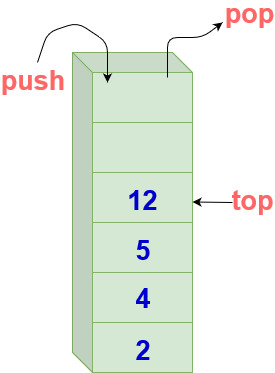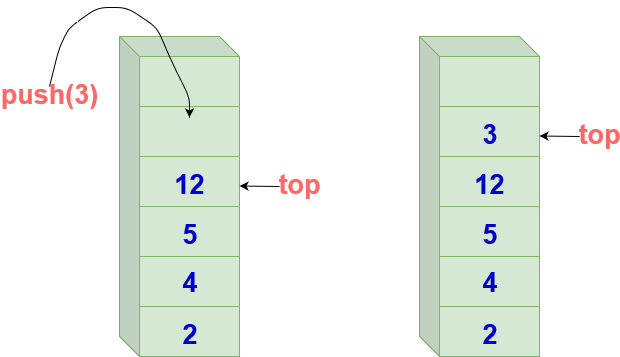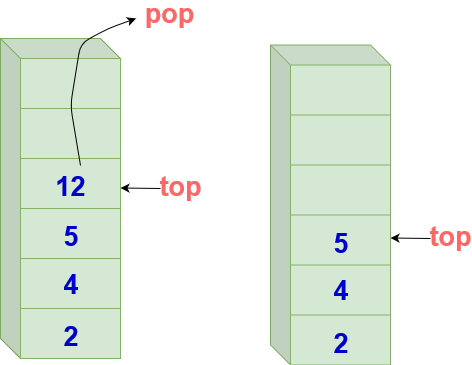# Complete Guide To Stacks

## Complete Guide To Stacks

Stack is a linear data structure that follows LIFO (Last In, First Out) or FILO (First In, Last Out) principle. It is an abstract data type of predefined capacity in which elements can be removed and added in a specific order.Insertion and deletion happen at the same end in stack data structure.

Visualise stack as a pile of plates in a restaurant.
Only the topmost plate is accessible.
To access any other plate, the plates on top of that plate need to be removed first.
The plate at the bottom of the pile can only be removed at the last after all plates on top of it have been removed.

## Array Implementation of Stacks

Array can be used to implement stack data structure. Only one element of a stack can be accessed at a time. This element is located at the index top.

``````class Stack{
int arr
int size
int top
};
``````

Array can be of any datatype.
Constructor to initialize size and top :

``````        Stack(int n)
{
size = n
top = -1 //denotes that stack is empty
}
``````

## Operations on Stacks

### Push Operation

Push operation adds an item in the stack. If the stack is full, then it is said to Overflow .
When a new element is inserted on top, the value of top increases by 1

1. Check if the stack is full before insertion.
2. If it is full, then display error and exit.
3. If the stack is not full, then increment the index on adding an element.3 is added to the stack after push operation

Code

``````void push()
{
if(top == size-1)// Check if the index is beyond the stack size
{
print(”Stack Overflow")
return
}
top=top+1//Increment the index on adding an element
s[top]=item// Add the element into the stack (array) as pointed by the index }
``````

### Pop Operation

Pop operation removes an item from the stack. The items are popped in the reversed order in which they are pushed. If the stack is empty, then it is said to be an Underflow condition.

1. Check if the stack index is beyond the initial index
2. Return a value -1 if the stack is empty
3. If the stack is not empty, return the topmost element12 is removed from the top of the stack by performing pop operation

Code

``````//operation returns the removed element
int pop()
{
if(top== -1) //Check if the stack index is beyond the initial index
{
print(”Empty Stack")
return -1 //Return a value -1 if the stack is empty
}
return s[top--] // Return the topmost element
}
``````

### isEmpty

Returns true if stack is empty, else false.

``````   bool isEmpty()
{
if(top==-1)
return true// stack empty
return false
}
``````

### Top or peek

Returns top element of stack.

``````int top()
{
if (top==-1)
{
print( "Stack is empty!")
return -1
}
else
return arr[top]
}
``````

### Time Complexities

Operation Time Complexity
Push O(1)
Pop O(1)
Top O(1)
Search O(n)

Scroll to Top